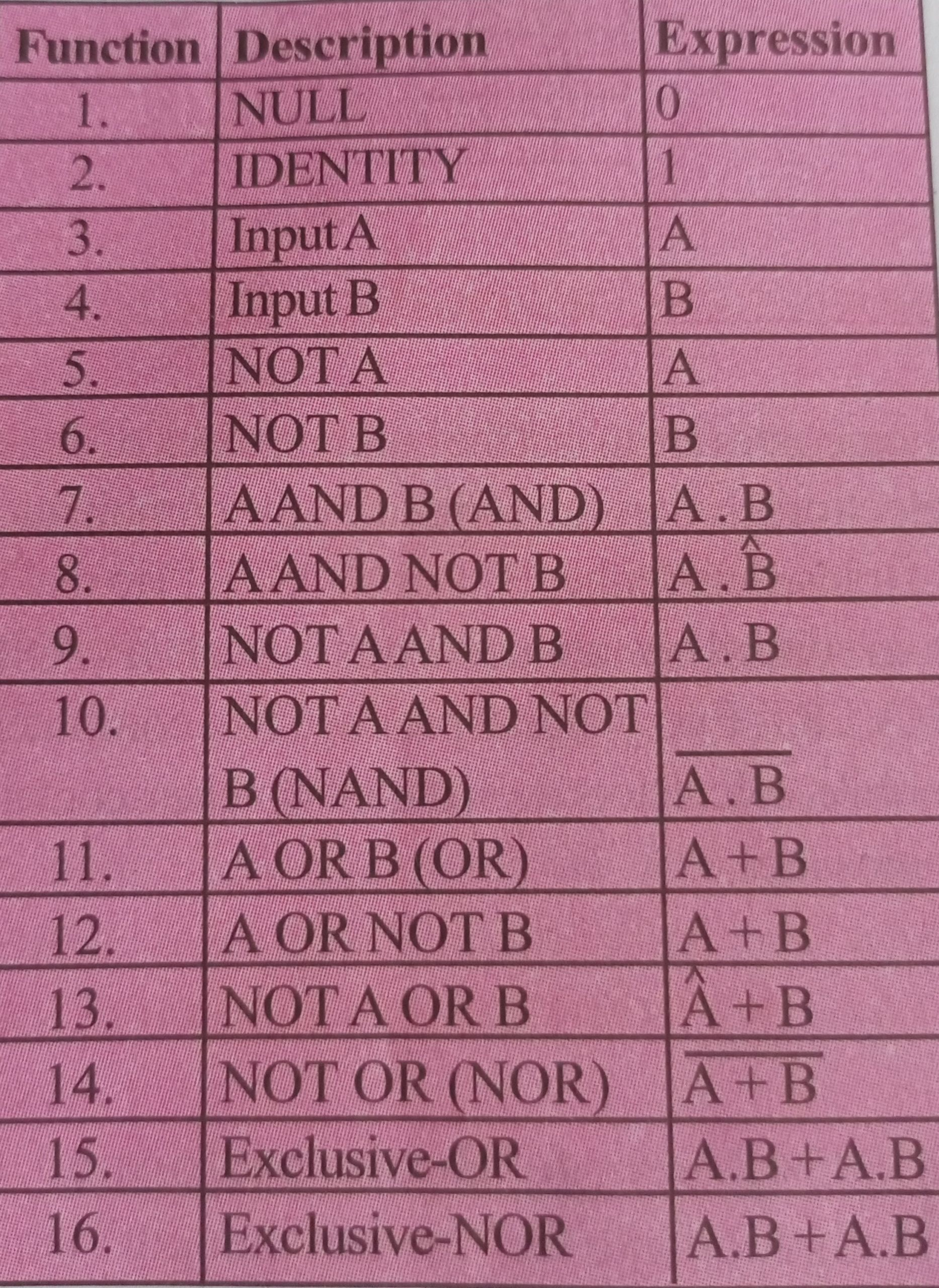Home » STANDARD SINGLE LOGIC GATE

# STANDARD SINGLE LOGIC GATELOGIC CIRCUIT 1 (STANDARD SINGLE LOGIC GATE)

Logic gate is a computer circuit with several inputs but one output that can be activated by particular combinations of inputs. A logic gate is an elementary building block of a digital circuit. Most logic gates have two inputs and one output. At any given moment, every terminal is in one of the two binary conditions low (0) or high (1), represented by different voltage levels. The logic state of a terminal can, and generally does, change often, as the circuit processes data. In most logic gates, the low state is approximately zero volts (0 V), while the high state is approximately five volts positive (+5 V). Logic gates are the building blocks of combinational logic circuits.

LOGIC GATE TRUTH TABLES

The input and output information of any Logic Gate or circuit can be plotted into a table to give a visual representation of the switching function of the system and this is commonly called a Truth Table. A logic gate truth table shows each possible input combination to the gate or circuit with the resultant output depending upon the combination of these input(s).

For example, consider a single 2-input logic circuit with input variables labelled as A and B. There are “four” possible input combinations or 2² of “OFF” and “ON” for the two inputs. However, when dealing with Boolean expressions and especially logic gate truth tables, we do not generally use “ON” or “OFF” but instead give them bit values which represent a logic level “1” or a logic level “0” respectively.

Then the four possible combinations of A and B for a 2-input logic gate is given as:

• Input Combination 1. – “OFF”- “OFF” or ( 0 0 )
• Input Combination 2. – “OFF”- “ON” or ( 0 1 )
• Input Combination 3. – “ON” – “OFF” or ( 1 0 )
• Input Combination 4. – “ON”- “ON” or ( 1 1 ).

Therefore, a 3-input logic circuit would have 8 possible input combinations or 2³ and a 4-input logic circuit would have 16 or 2 raised to power 4, and so on as the number of inputs increases. Then a logic circuit with “n” number of inputs would have 2 raised to power “n” possible input combinations of both “OFF” and “ON”. In order to keep things simple to understand, we will only deal with simple 2-input logic gates, but the principals are still the same for gates with more inputs.

CLASSIFICATION OF LOGIC CIRCUITS

Logic circuits can be classified into two. They are:

• Standard Single Logic Gate.
• Alternative Logic Gate.

Standard Single Logic Gate

The standard single logic gates are AND, OR and NOT (Inverter).

1) AND gate: The AND gate is so named because, if 0 is called “false” and 1 is called “true,” the gate acts in the same way as the logical “and” operator. The following illustration and table show the circuit symbol and logic combinations for an AND gate. In the symbol, the input terminals are at left and the output terminal is at right. The output is “true” when both inputs are “true.” Otherwise, the output is false.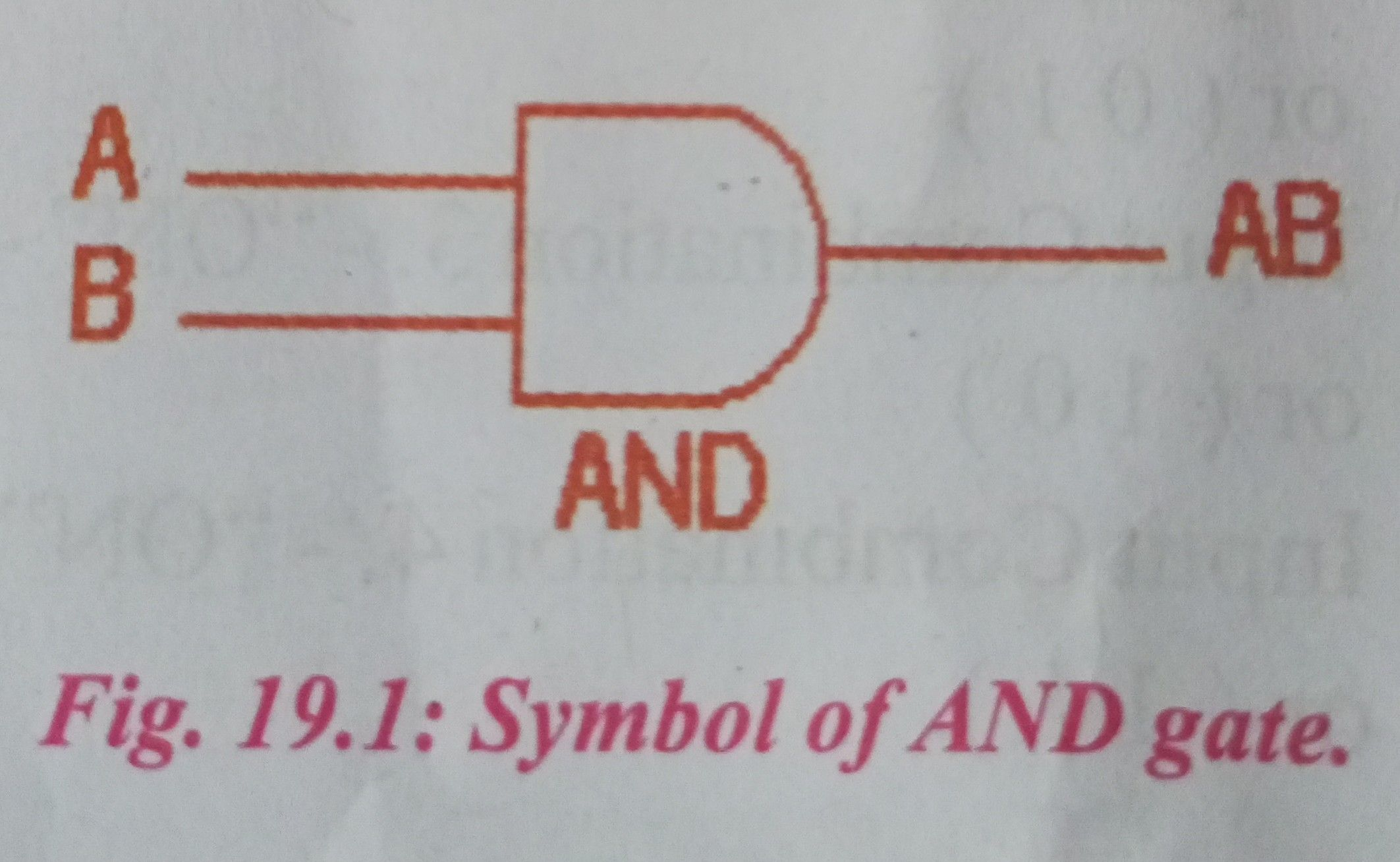The AND gate is an electronic circuit that gives a high output (1) only if all its inputs are high. A dot (.) is used to show the AND operation i.e. A.B. Bear in mind that this dot is sometimes omitted i.e. AB. AND gate operation can be shown from the truth table below.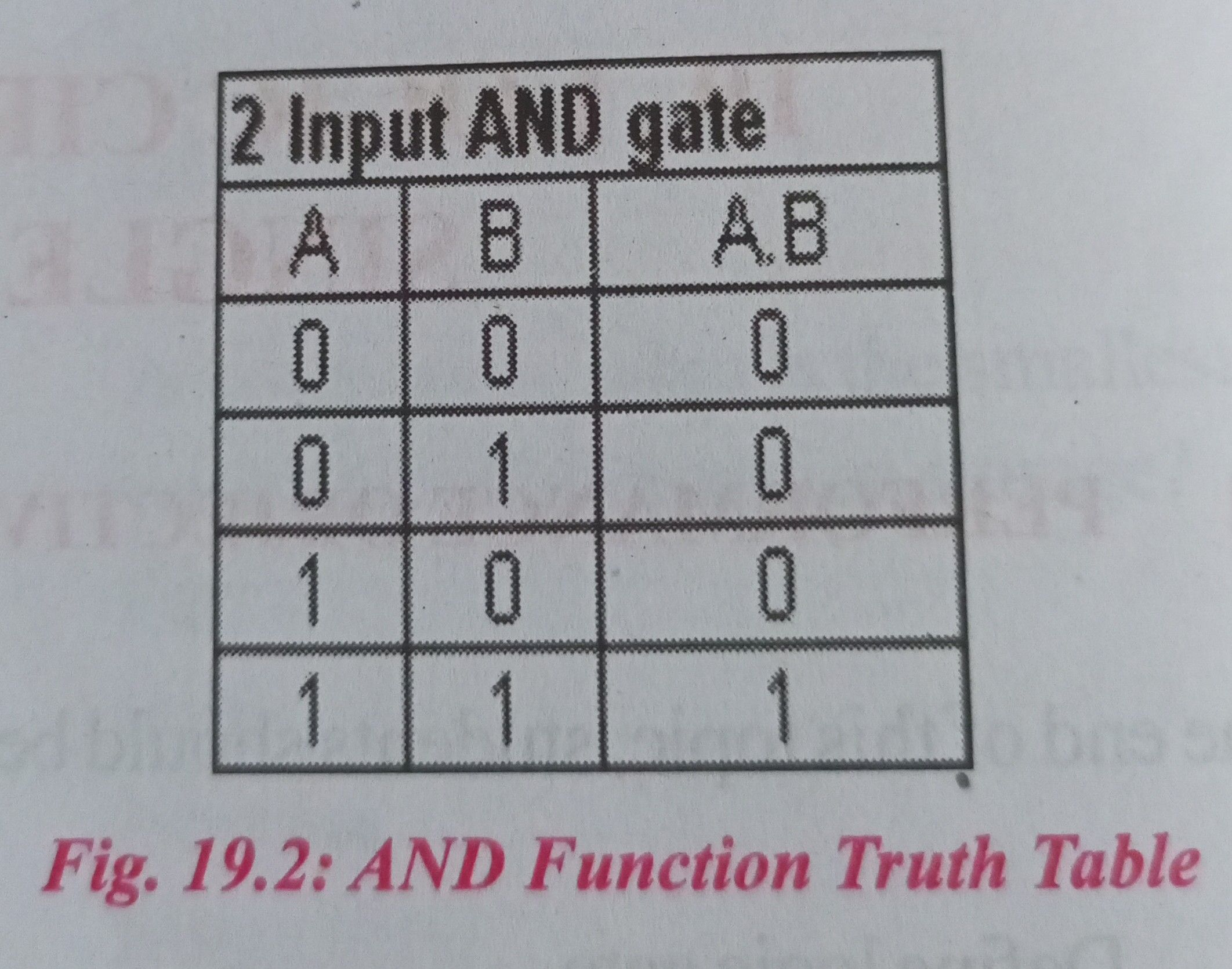On a basic electrical circuit making an AND gate using just switches, a lamp and power supply, it has just switches in series with a lamp. All the switches must be on for the lamp to go.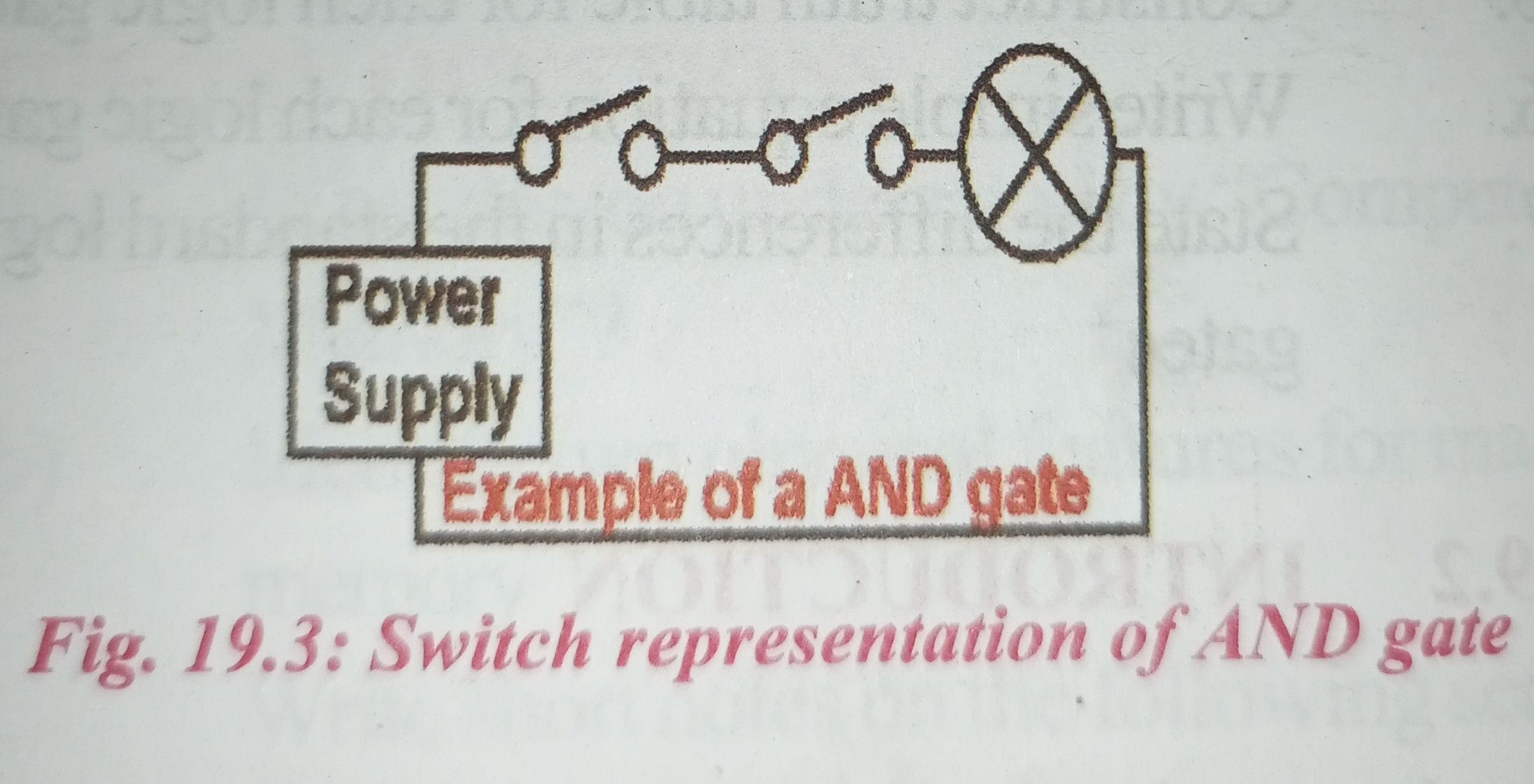2) OR gate: The OR gate gets its name from the fact that it behaves after the fashion of the logical inclusive “or.” The output is “true” if either or both of the inputs are “true.” If both inputs are “false,” then the output is “false.”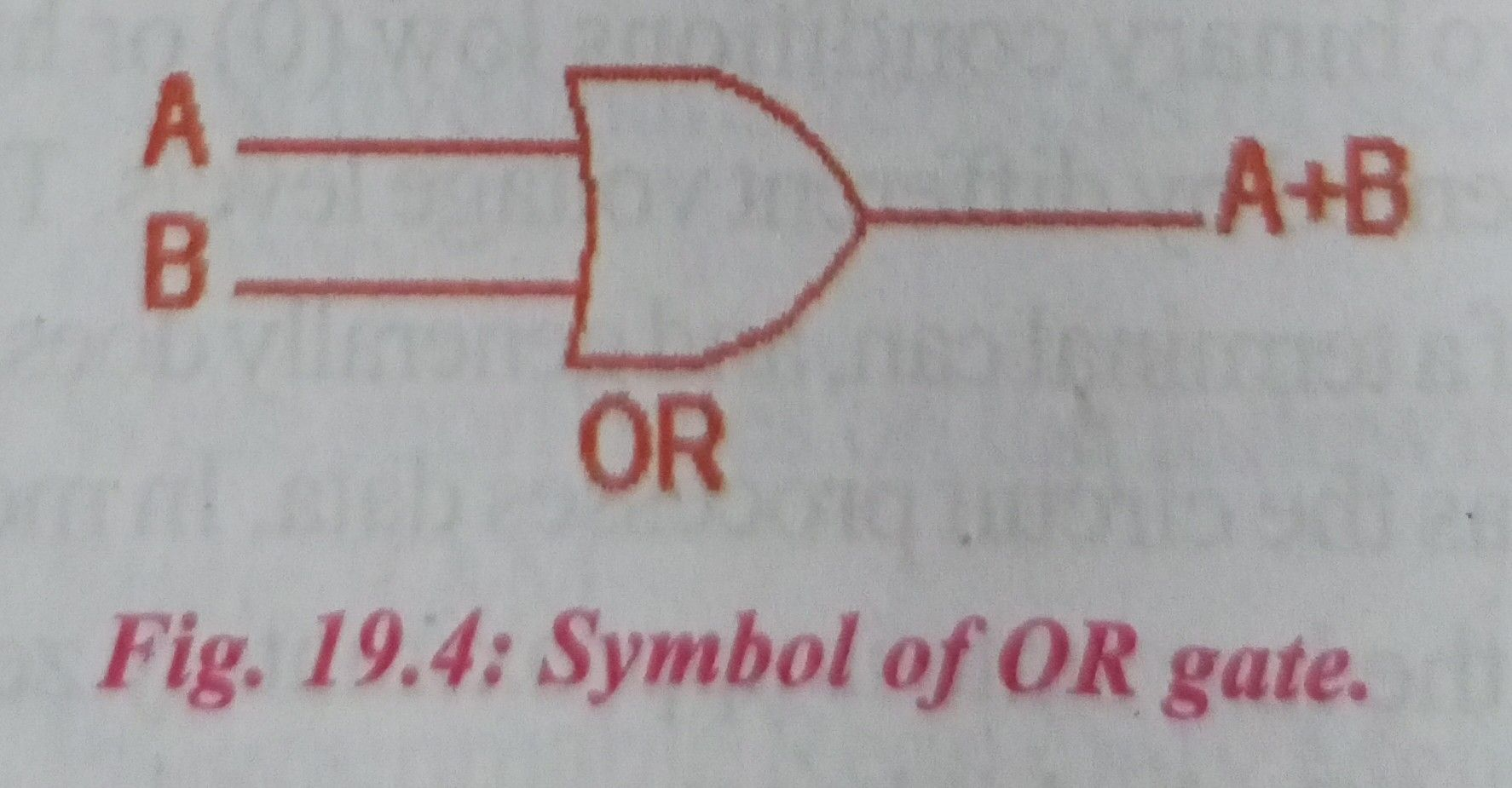The OR gate is an electronic circuit that gives a high output (1) if one or more of its inputs are high. A plus (+) is used to show the OR operation. OR gate operation can be shown from the truth table below.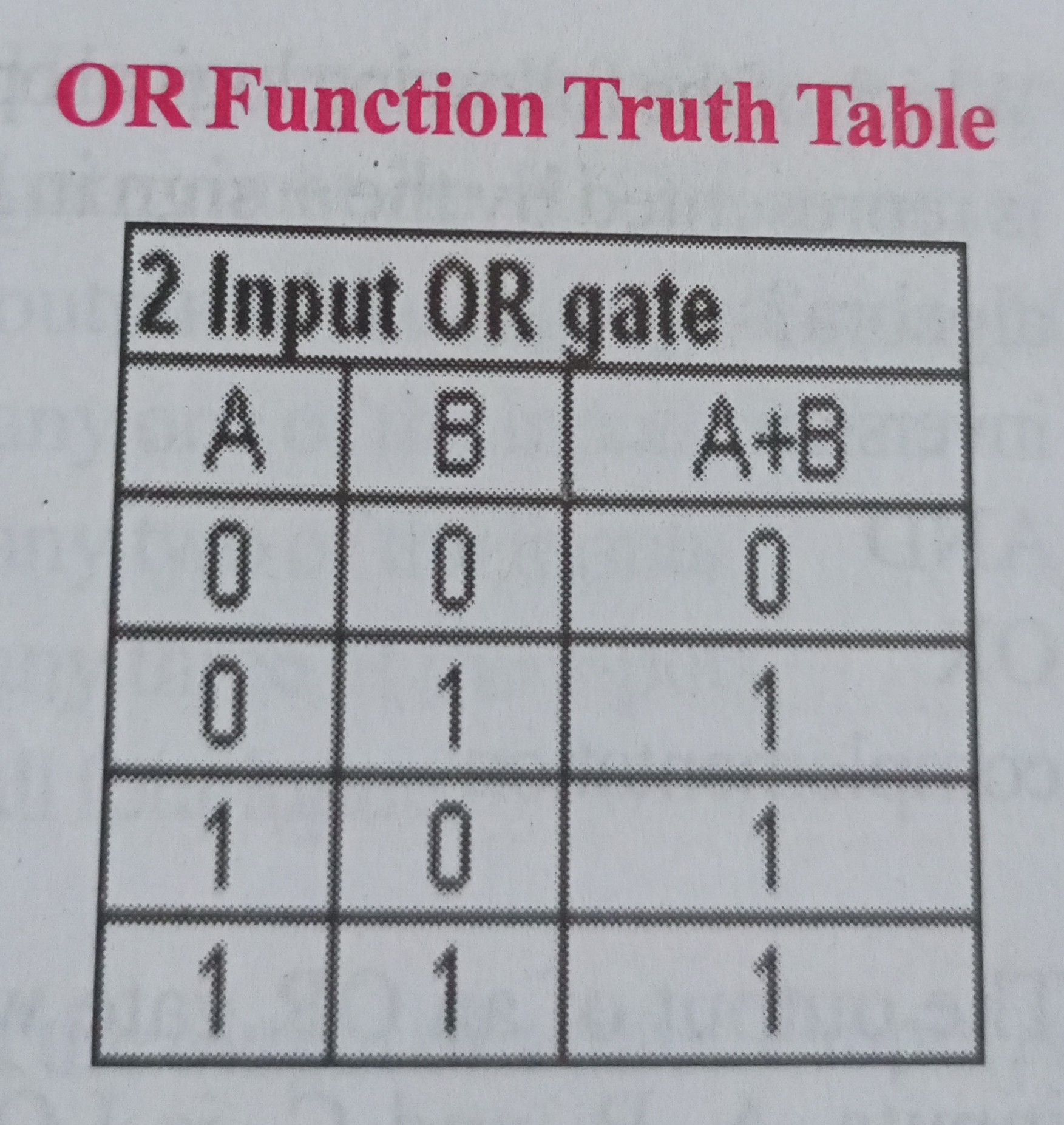On a basic electrical circuit making OR gate using just switches, a lamp and power supply, this has all the switches in parallel with each other and then in series with a lamp. Any one of the switches closed (or turned on) can turn the lamp on.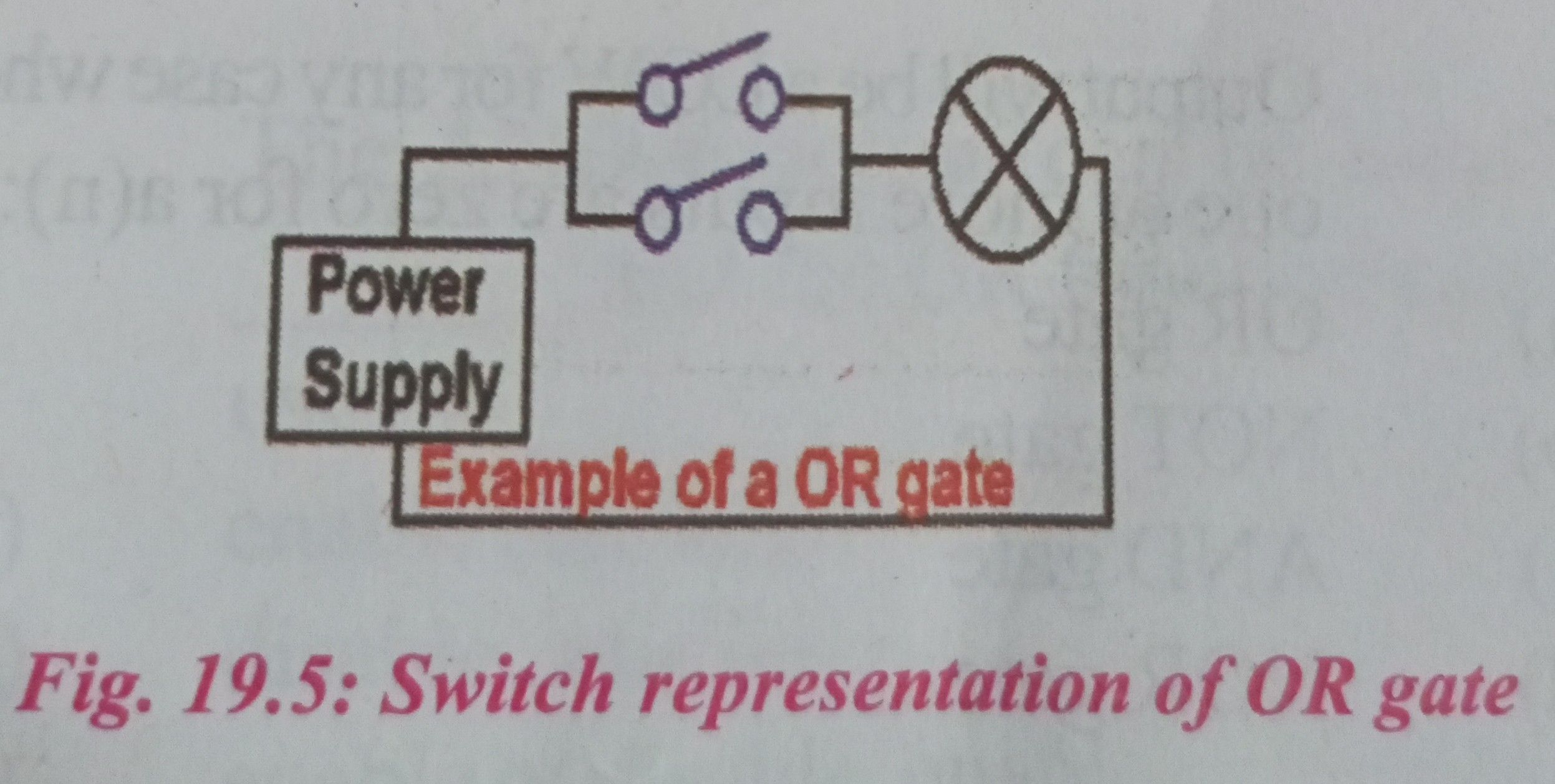3) NOT gate (Inverter): The NOT gate is an electronic circuit that produces an inverted version of the input at its output. It is also known as an inverter. It reverses the logic state.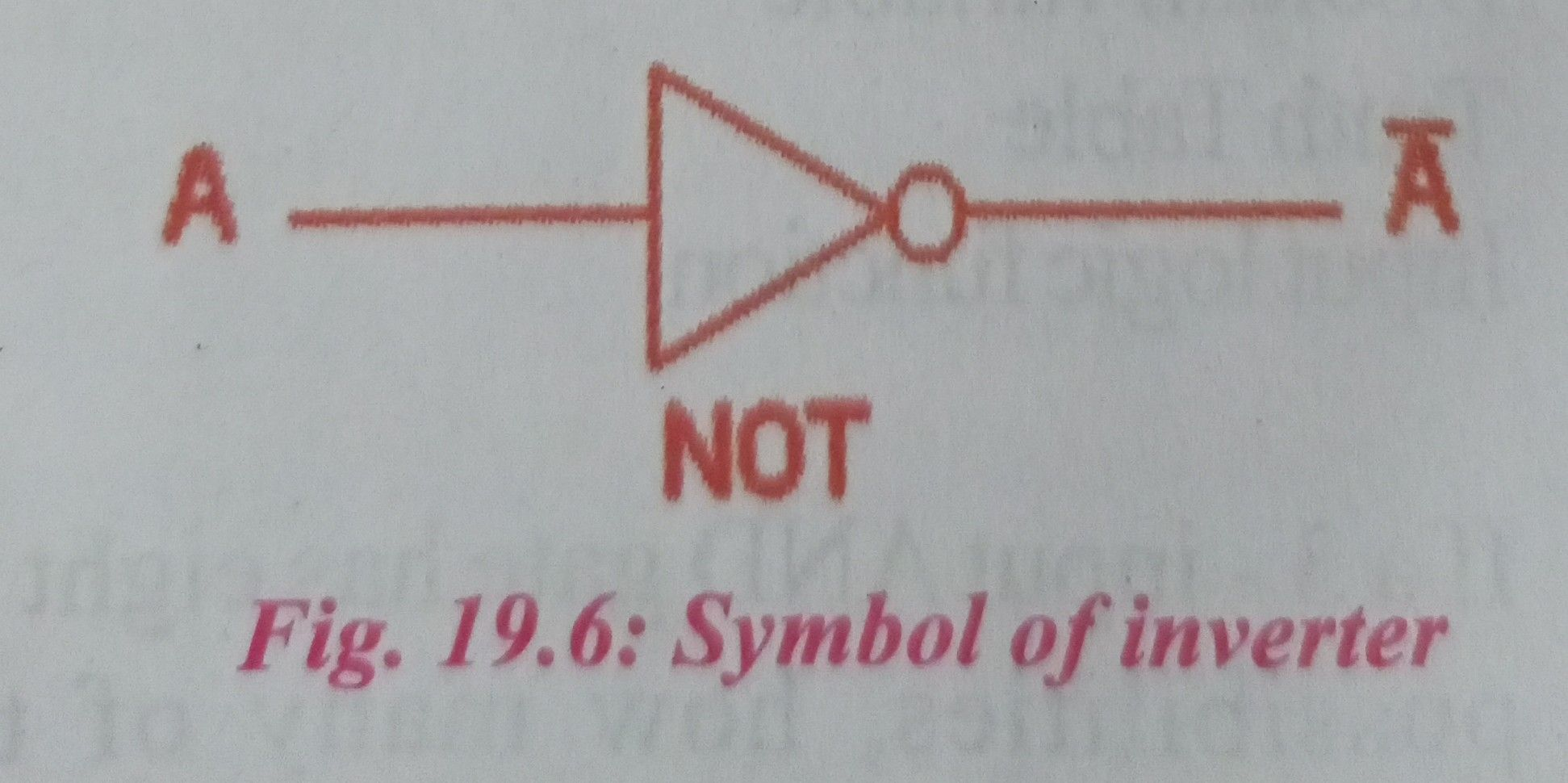If the input variable is A, the inverted output is known as NOT A. This is also shown as A’, or A with a bar over the top, as shown at the outputs. The diagrams below show two ways that the NAND logic gate can be configured to produce a NOT gate. It can also be done using NOR logic gates in the same way.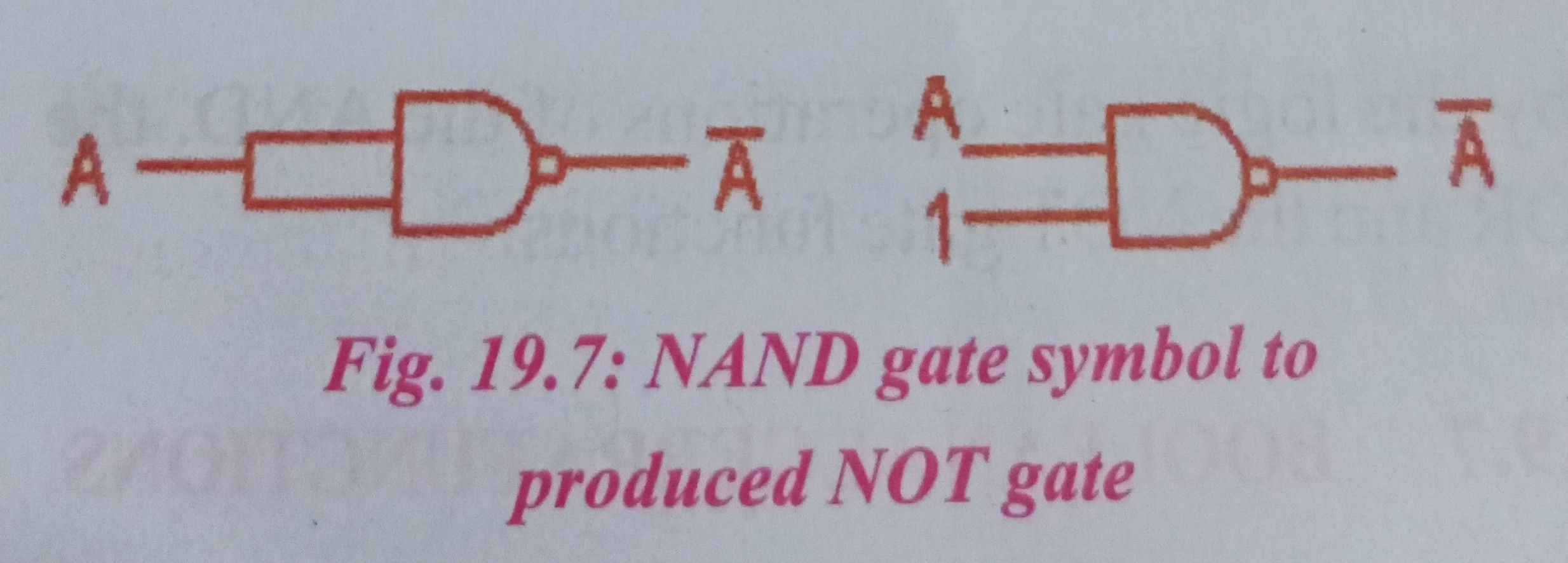NOT gate operation can be shown from the truth table below.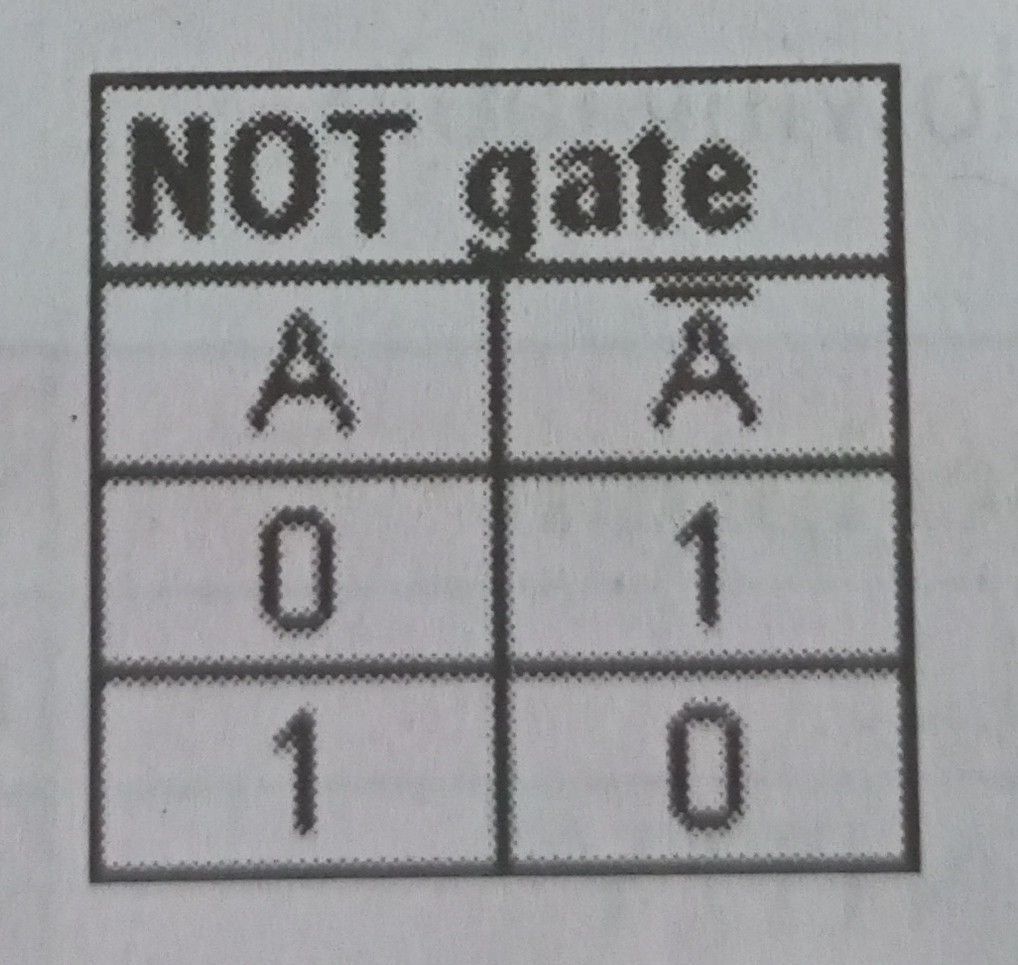USES OF LOGIC GATES

Logic gates are in fact the building block of digital electronics; they are formed by the combination of transistors (either BJT or MOSFET) to realize some digital operations such as logical OR, AND, INVERT. Every digital product, like computers, mobile, calculators even digital watches, contains logic gates.

INTRODUCTION TO BOOLEAN

In 1854, George Boole performed an investigation into the “laws of thought” which were based on a simplified version of the “group” or “set” theory, and from this Boolean or “Switching” algebra was developed. Boolean Algebra deals mainly with the theory that both logic and set operations are either “TRUE” or “FALSE” but not both at the same time.

For example, A + A = A and not 2A as it would be in normal algebra. Boolean algebra is a simple and effective way of representing the switching action of standard Logic Gates and the basic logic statements which concern us here are given by the logic gate operations of the AND, the OR and the NOT gate functions.

BOOLEAN ALGEBRA FUNCTIONS

Simple 2-input AND, OR and NOT Gates can be represented by 16 possible functions as shown in the following table.